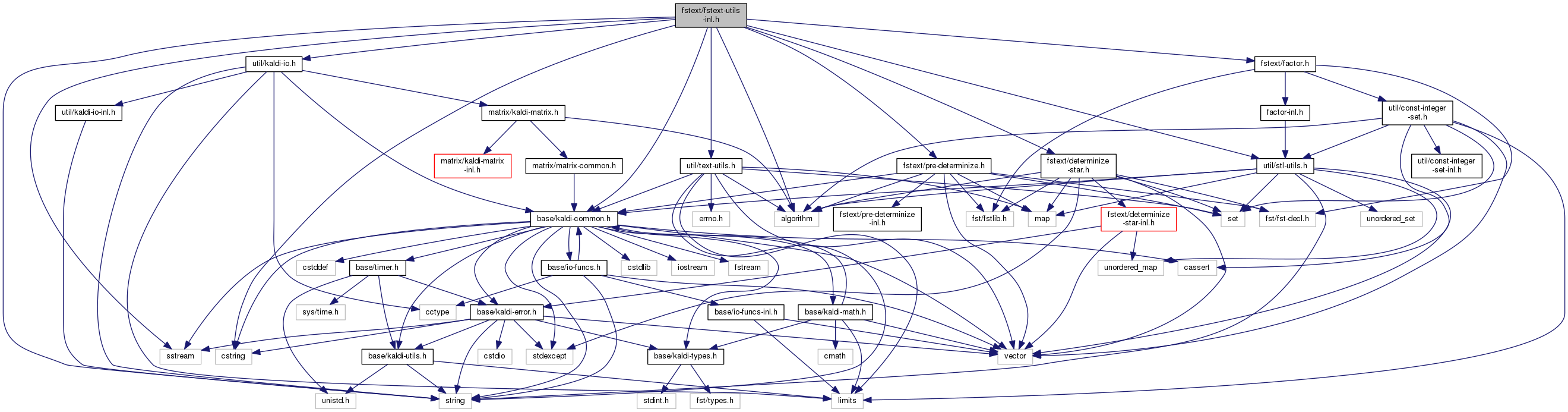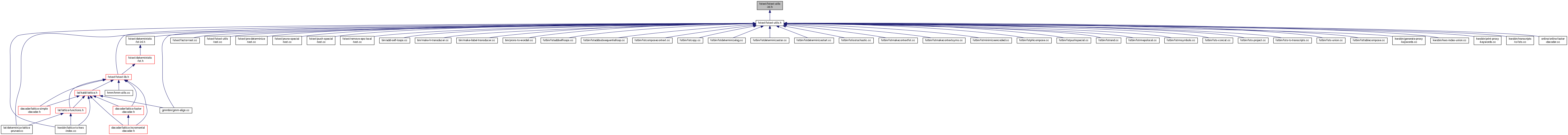fstext-utils-inl.h File Reference
`#include <cstring>`
`#include "base/kaldi-common.h"`
`#include "util/stl-utils.h"`
`#include "util/text-utils.h"`
`#include "util/kaldi-io.h"`
`#include "fstext/factor.h"`
`#include "fstext/pre-determinize.h"`
`#include "fstext/determinize-star.h"`
`#include <sstream>`
`#include <algorithm>`
`#include <string>`
Include dependency graph for fstext-utils-inl.h:This graph shows which files directly or indirectly include this file:Go to the source code of this file.

## Classes

class  MapInputSymbolsMapper< Arc, I >

struct  IdentityFunction< T >

## Namespaces

fst
For an extended explanation of the framework of which grammar-fsts are a part, please see Support for grammars and graphs with on-the-fly parts. (i.e.

## Functions

template<class Arc >
Arc::Label HighestNumberedOutputSymbol (const Fst< Arc > &fst)
Returns the highest numbered output symbol id of the FST (or zero for an empty FST. More...

template<class Arc >
Arc::Label HighestNumberedInputSymbol (const Fst< Arc > &fst)
Returns the highest numbered input symbol id of the FST (or zero for an empty FST. More...

template<class Arc >
Arc::StateId NumArcs (const ExpandedFst< Arc > &fst)
Returns the total number of arcs in an FST. More...

template<class Arc , class I >
void GetOutputSymbols (const Fst< Arc > &fst, bool include_eps, std::vector< I > *symbols)
GetOutputSymbols gets the list of symbols on the output of fst (including epsilon, if include_eps == true) More...

template<class Arc , class I >
void GetInputSymbols (const Fst< Arc > &fst, bool include_eps, std::vector< I > *symbols)
GetInputSymbols gets the list of symbols on the input of fst (including epsilon, if include_eps == true), as a sorted, unique list. More...

template<class Arc , class I >
void RemoveSomeInputSymbols (const std::vector< I > &to_remove, MutableFst< Arc > *fst)
RemoveSomeInputSymbols removes any symbol that appears in "to_remove", from the input side of the FST, replacing them with epsilon. More...

template<class Arc , class I >
void MapInputSymbols (const std::vector< I > &symbol_mapping, MutableFst< Arc > *fst)

template<class Arc , class I >
bool GetLinearSymbolSequence (const Fst< Arc > &fst, std::vector< I > *isymbols_out, std::vector< I > *osymbols_out, typename Arc::Weight *tot_weight_out)
GetLinearSymbolSequence gets the symbol sequence from a linear FST. More...

template<class Arc >
void ConvertNbestToVector (const Fst< Arc > &fst, std::vector< VectorFst< Arc > > *fsts_out)
This function converts an FST with a special structure, which is output by the OpenFst functions ShortestPath and RandGen, and converts them into a std::vector of separate FSTs. More...

template<class Arc >
void NbestAsFsts (const Fst< Arc > &fst, size_t n, std::vector< VectorFst< Arc > > *fsts_out)
Takes the n-shortest-paths (using ShortestPath), but outputs the result as a vector of up to n fsts. More...

template<class Arc , class I >
void MakeLinearAcceptorWithAlternatives (const std::vector< std::vector< I > > &labels, MutableFst< Arc > *ofst)
Creates an unweighted acceptor with a linear structure, with alternatives at each position. More...

template<class Arc , class I >
void MakeLinearAcceptor (const std::vector< I > &labels, MutableFst< Arc > *ofst)
Creates unweighted linear acceptor from symbol sequence. More...

template<class I >
void GetSymbols (const SymbolTable &symtab, bool include_eps, std::vector< I > *syms_out)

template<class Arc >
void SafeDeterminizeWrapper (MutableFst< Arc > *ifst, MutableFst< Arc > *ofst, float delta=kDelta)
Does PreDeterminize and DeterminizeStar and then removes the disambiguation symbols. More...

template<class Arc >
void SafeDeterminizeMinimizeWrapper (MutableFst< Arc > *ifst, VectorFst< Arc > *ofst, float delta=kDelta)
SafeDeterminizeMinimizeWapper is as SafeDeterminizeWrapper except that it also minimizes (encoded minimization, which is safe). More...

void DeterminizeStarInLog (VectorFst< StdArc > *fst, float delta, bool *debug_ptr, int max_states)

void DeterminizeInLog (VectorFst< StdArc > *fst)

void SafeDeterminizeMinimizeWrapperInLog (VectorFst< StdArc > *ifst, VectorFst< StdArc > *ofst, float delta=kDelta)
SafeDeterminizeMinimizeWapperInLog is as SafeDeterminizeMinimizeWrapper except it first casts tothe log semiring. More...

void SafeDeterminizeWrapperInLog (VectorFst< StdArc > *ifst, VectorFst< StdArc > *ofst, float delta)

template<class Arc >
void RemoveWeights (MutableFst< Arc > *ifst)

template<class Arc >
bool PrecedingInputSymbolsAreSame (bool start_is_epsilon, const Fst< Arc > &fst)
Returns true if and only if the FST is such that the input symbols on arcs entering any given state all have the same value. More...

template<class Arc , class F >
bool PrecedingInputSymbolsAreSameClass (bool start_is_epsilon, const Fst< Arc > &fst, const F &f)
This is as PrecedingInputSymbolsAreSame, but with a functor f that maps labels to classes. More...

template<class Arc >
bool FollowingInputSymbolsAreSame (bool end_is_epsilon, const Fst< Arc > &fst)
Returns true if and only if the FST is such that the input symbols on arcs exiting any given state all have the same value. More...

template<class Arc , class F >
bool FollowingInputSymbolsAreSameClass (bool end_is_epsilon, const Fst< Arc > &fst, const F &f)

template<class Arc >
void MakePrecedingInputSymbolsSame (bool start_is_epsilon, MutableFst< Arc > *fst)
MakePrecedingInputSymbolsSame ensures that all arcs entering any given fst state have the same input symbol. More...

template<class Arc , class F >
void MakePrecedingInputSymbolsSameClass (bool start_is_epsilon, MutableFst< Arc > *fst, const F &f)
As MakePrecedingInputSymbolsSame, but takes a functor object that maps labels to classes. More...

template<class Arc >
void MakeFollowingInputSymbolsSame (bool end_is_epsilon, MutableFst< Arc > *fst)
MakeFollowingInputSymbolsSame ensures that all arcs exiting any given fst state have the same input symbol. More...

template<class Arc , class F >
void MakeFollowingInputSymbolsSameClass (bool end_is_epsilon, MutableFst< Arc > *fst, const F &f)
As MakeFollowingInputSymbolsSame, but takes a functor object that maps labels to classes. More...

template<class Arc >
VectorFst< Arc > * MakeLoopFst (const std::vector< const ExpandedFst< Arc > * > &fsts)
MakeLoopFst creates an FST that has a state that is both initial and final (weight == Weight::One()), and for each non-NULL pointer fsts[i], it has an arc out whose output-symbol is i and which goes to a sub-graph whose input language is equivalent to fsts[i], where the final-state becomes a transition to the loop-state. More...

template<class Arc >
void ClearSymbols (bool clear_input, bool clear_output, MutableFst< Arc > *fst)
ClearSymbols sets all the symbols on the input and/or output side of the FST to zero, as specified. More...

template<class Arc >
void ApplyProbabilityScale (float scale, MutableFst< Arc > *fst)
ApplyProbabilityScale is applicable to FSTs in the log or tropical semiring. More...

template<class Arc >
ssize_t FindSelfLoopWithILabel (const Fst< Arc > &fst, typename Arc::StateId s)

template<class Arc >
bool EqualAlign (const Fst< Arc > &ifst, typename Arc::StateId length, int rand_seed, MutableFst< Arc > *ofst, int num_retries=10)
EqualAlign is similar to RandGen, but it generates a sequence with exactly "length" input symbols. More...

template<class Arc >
void RemoveUselessArcs (MutableFst< Arc > *fst)

template<class Arc >
void PhiCompose (const Fst< Arc > &fst1, const Fst< Arc > &fst2, typename Arc::Label phi_label, MutableFst< Arc > *ofst)

template<class Arc >
void PropagateFinalInternal (typename Arc::Label phi_label, typename Arc::StateId s, MutableFst< Arc > *fst)

template<class Arc >
void PropagateFinal (typename Arc::Label phi_label, MutableFst< Arc > *fst)

template<class Arc >
void RhoCompose (const Fst< Arc > &fst1, const Fst< Arc > &fst2, typename Arc::Label rho_label, MutableFst< Arc > *ofst)

template<>
bool IsStochasticFst (const Fst< LogArc > &fst, float delta, LogArc::Weight *min_sum, LogArc::Weight *max_sum)

template<class Arc >
bool IsStochasticFst (const Fst< Arc > &fst, float delta=kDelta, typename Arc::Weight *min_sum=NULL, typename Arc::Weight *max_sum=NULL)
This function returns true if, in the semiring of the FST, the sum (within the semiring) of all the arcs out of each state in the FST is one, to within delta. More...

bool IsStochasticFstInLog (const Fst< StdArc > &fst, float delta, StdArc::Weight *min_sum, StdArc::Weight *max_sum)